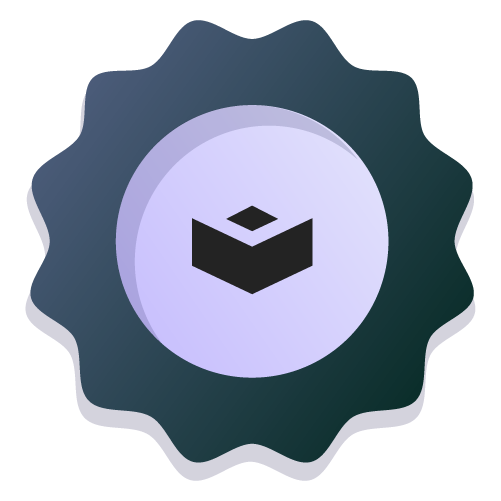Introduction to Supervised Learning with scikit-learn

# Linear regression

In this project, you'll practice a simple regression model. You will evaluate the fundamentals of regression modeling using scikit-learn.

## Project Activities

All our Data Science projects include bite-sized activities to test your knowledge and practice in an environment with constant feedback.

All our activities include solutions with explanations on how they work and why we chose them.

codevalidated

### Use train_test_split to split the data into training and testing sets. Split the dataset in 80% training, 20% testing, and random_state=0.

Set the random_state parameter to a desired integer value for reproducibility. Store this variable in `random_state` and then used in the function.

Store the values in the variables in `X_train`,`X_test`,`y_train` and `y_test`.

codevalidated

### Linea Regression

Create an instance of the `LinearRegression` and store the model in `lr`.

codevalidated

### Train the linear regression model

It's time to train the linear regression model using the training dataset.

codevalidated

### Make predictions on the test set

Use the trained model to make predictions on the test data. Store the prediction in `y_pred`.Author

#### Verónica Barraza

This project is part of

## Introduction to Supervised Learning with scikit-learn

Explore other projectsAnurag VermaAnurag VermaSantiago Basulto